# Multiple Choice Questions and Answers on Digital Electronics

## Multiple Choice Questions and Answers on Digital Electronics

In addition to reading the questions and answers on my site, I would suggest you to check the following, on amazon, as well:

Q1. The binary number 10101 is equivalent to decimal number …………..

1. 19
2. 12
3. 27
4. 21

Q2. The universal gate is ………………

1. NAND gate
2. OR gate
3. AND gate
4. None of the above

Q3. The inverter is ……………

1. NOT gate
2. OR gate
3. AND gate
4. None of the above

Q4. The inputs of a NAND gate are connected together. The resulting circuit is ………….

1. OR gate
2. AND gate
3. NOT gate
4. None of the above

Q5. The NOR gate is OR gate followed by ………………

1. AND gate
2. NAND gate
3. NOT gate
4. None of the above

Q6. The NAND gate is AND gate followed by …………………

1. NOT gate
2. OR gate
3. AND gate
4. None of the above

Q7. Digital circuit can be made by the repeated use of ………………

1. OR gates
2. NOT gates
3. NAND gates
4. None of the above

Q8. The only function of NOT gate is to ……………..

1. Stop signal
2. Invert input signal
3. Act as a universal gate
4. None of the above

Q9. When an input signal 1 is applied to a NOT gate, the output is ………………

1. 0
2. 1
3. Either 0 & 1
4. None of the above

Q10. In Boolean algebra, the bar sign (-) indicates ………………..

1. OR operation
2. AND operation
3. NOT operation
4. None of the above

Q11. The resolution of an n bit DAC with a maximum input of 5 V is 5 mV. The value of n is …….

1. 8
2. 9
3. 10
4. 11

Explanation:

(5/2N-1)1000 = 5 or N = 10

Q12. 2’s complement of binary number 0101 is ………..

1. 1011
2. 1111
3. 1101
4. 1110

Explanation: 1’s complement of 0101 is 1010 and 2’s complement is 1010+1 = 1011.

Q13. An OR gate has 4 inputs. One input is high and the other three are low. The output is …….

1. Low
2. High
3. alternately high and low
4. may be high or low depending on relative magnitude of inputs

Explanation: In OR any input high means high output.

Q14. Decimal number 10 is equal to binary number ……………

1. 1110
2. 1010
3. 1001
4. 1000

Explanation: 1010 = 8 + 2 = 10 in decimal.

Q15. Both OR and AND gates can have only two inputs.

1. True
2. False

Explanation:  Any number of inputs are possible.

Q16. A device which converts BCD to seven segments is called ……..

1. Encoder
2. Decoder
3. Multiplexer
4. None of these

Explanation:  Decoder converts binary/BCD to alphanumeric.

Q17. In 2’s complement representation the number 11100101 represents the decimal number ……………

1. +37
2. -31
3. +27
4. -27

Explanation:

A = 11100101. Therefore Ā = 00011010 and A’ = Ā + 1 = 00011011 = 16 + 8 + 2 + 1 = 27. Therefore A = -27.

Q18. A decade counter skips ………..

1. binary states 1000 to 1111
2. binary states 0000 to 0011
3. binary states 1010 to 1111
4. binary states 1111 to higher

Explanation:  A decade counter counts from 0 to 9. It has 4 flip-flops. The states skipped are 10 to 15 or 1010 to 1111.

Q19. BCD input 1000 is fed to a 7 segment display through a BCD to 7 segment decoder/driver. The segments which will lit up are ………….

1. a, b, d
2. a, b, c
3. all
4. a, b, g, c, d

Explanation:  1000 equals decimal 8 Therefore all segments will lit up.

Q20. A ring counter with 5 flip flops will have ………. states.

1. 5
2. 10
3. 32
4. Infinite

Q21. For the gate in the given figure the output will be ………..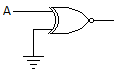1. 0
2. 1
3. A
4. Ā

Explanation:  If A = 0, Y = 1 and A = 1, Y = 0 Therefore Y = Ā.

Q22. In the expression A + BC, the total number of minterms will be ………

1. 2
2. 3
3. 4
4. 5

Q23. The circuit in the given figure is a ………… gate.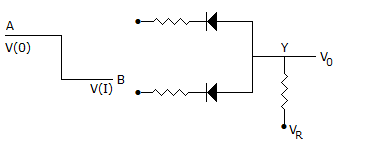1. positive logic OR gate
2. negative logic OR gate
3. negative logic AND gate
4. positive logic AND gate

Explanation:  Since V(1) is lower state than V(0) it is a negative logic circuit. Since diodes are in parallel, it is an OR gate.

Q24. Which of the following is non-saturating?

1. TTL
2. CMOS
3. ECL
4. Both 1 and 2

Q25. The number of digits in octal system is ………

1. 8
2. 7
3. 9
4. 10

Explanation:  The octal system has 8 digits 0 to 7.

Q16.

Explanation:

Q26. The access time of a word in 4 MB main memory is 100 ms. The access time of a word in a 32 kb data cache memory is 10 ns. The average data cache bit ratio is 0.95. The efficiency of memory access time is ………

1. 9.5 ns
2. 14.5 ns
3. 20 ns
4. 95 ns

Explanation:  Access time = 0.95 x 10 + 0.05 x 100.

Q27. The expression Y = pM (0, 1, 3, 4) is …………..

1. POS
2. SOP
3. Hybrid
4. none of these

Explanation:  This is a product of sums expression.

Q28. An 8 bit DAC has a full scale output of 2 mA and full scale error of ± 0.5%. If input is 10000000 the range of outputs is ………….

1. 994 to 1014 μA
2. 990 to 1020 μA
3. 800 to 1200 μA
4. none of the above

Explanation: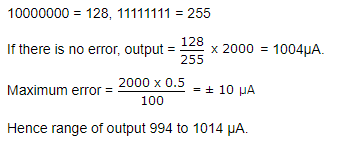Q29. Decimal 43 in hexadecimal and BCD number system is respectively……. and ……..

1. B2 and 01000011
2. 2B and 01000011
3. 2B and 00110100
4. B2 and 01000100

Explanation: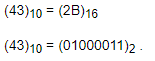Q30. The circuit of the given figure realizes the function …………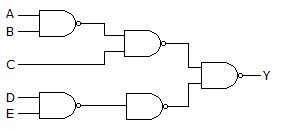Explanation: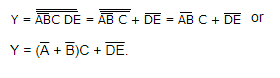Q31. An AND gate has two inputs A and B and one inhibit input 3, Output is 1 if

1. A = 1, B = 1, S = 1
2. A = 1, B = 1, S = 0
3. A = 1, B = 0, S = 1
4. A = 1, B = 0, S = 0

Explanation:  All AND inputs must be 1 and inhibit 0 for output to be 1.

Q32. The greatest negative number which can be stored is 8 bit computer using 2’s complement arithmetic is ……..

1. -256
2. -128
3. -255
4. -127

Explanation:  The largest negative number is 1000 0000 = -128.

Q33. A JK flip flop has tpd= 12 ns. The largest modulus of a ripple counter using these flip flops and operating at 10 MHz is ……..

1. 16
2. 64
3. 128
4. 256

Explanation: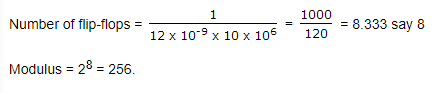Q34. The basic storage element in a digital system is ………….

1. flipflop
2. counter
3. multiplexer
4. encoder

Explanation: Storing can be done only in memory and flip-flop is a memory element.

Q35. In a ripple counter,

1. whenever a flipflop sets to 1, the next higher FF toggles
2. whenever a flipflop sets to 0, the next higher FF remains unchanged
3. whenever a flipflop sets to 1, the next higher FF faces race condition
4. whenever a flipflop sets to 0, the next higher FF faces race condition

Explanation:  In a ripple counter the effect ripples through the counter.

Q36. A 12 bit ADC is used to convert analog voltage of 0 to 10 V into digital. The resolution is ……….

1. 2.44 mV
2. 24.4 mV
3. 1.2 V
4. none of these

Explanation: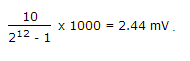Q37. For the truth table of the given figure Y = ………….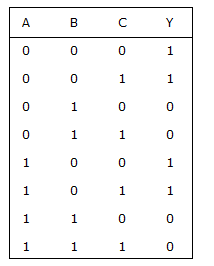1. A + B + C
2. Ā +BC
3. Ā

Explanation: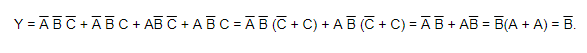2. two half adders and a OR gate
3. two half adders and a NOT gate

Q39. If the functions w, x, y, z are as follows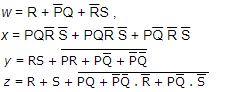1. w = z x = z
2. w = z, x = y
3. w = y
4. w = y = z

Q40. The output of a half adder is ……….

1. Sum
2. Sum and Carry
3. Carry
4. none of these

Q41. Minimum number of 2-input NAND gates required to implement the function F = (x + y) (Z + W) is ………..

1. 3
2. 4
3. 5
4. 6

Explanation: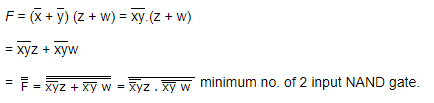Q42. Which device has one input and many outputs?

1. Multiplexer
2. Demultiplexer
3. Counter
4. Flip flop

Explanation: Demultiplexer takes data from one line and directs it to any of its N output depending on the status of its select lines.

1. it costs less
2. it is faster
3. it is more accurate
4. uses fewer gates

Explanation:

Q27.

Explanation: In look ahead carry adder the carry is directly derived from the gates when original inputs are being added. Hence the addition is fast. This process requires more gates and is costly.

Q44. The counter in the given figure is ………….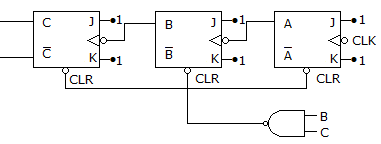1. Mod 3
2. Mod 6
3. Mod 8
4. Mod 7

Explanation: When counter is 110 the counter resets. Hence mod 6.

Q45. In register index addressing mode the effective address is given by ……..

1. index register value
2. sum of the index register value and the operand
3. operand
4. difference of the index register value and the operand

Explanation:

4 = 22, in up scaling digit will be shifted by two bit in right direction.

Q46. 7BF16 = __________ 2

1. 0111 1011 1110
2. 0111 1011 1111
3. 0111 1011 0111
4. 0111 1011 0011

Explanation:

7BF16 = 7 x 162 + 11 x 161 + 15 x 160 = 1983 in decimal = 0111 1011 1111 in binary.

Q47. For the minterm designation Y = ∑ m (1, 3, 5, 7) the complete expression is …………..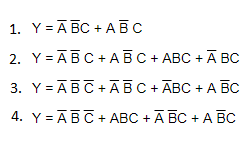Explanation: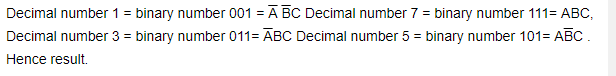Q48. Zero suppression is not used in actual practice.

1. True
2. False

Explanation: Zero suppression is commonly used.

Q49. A counter type A/D converter contains a 4 bit binary ladder and a counter driven by a 2 MHz clock. Then conversion time is ………..

1. 8 μ sec
2. 10 μ sec
3. 2 μ sec
4. 5 μ sec

Explanation: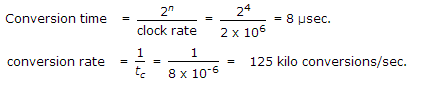Q50. The hexadecimal number (3E8)16 is equal to decimal number ………

1. 1000
2. 982
3. 768
4. 323

Explanation:  3 x 162 + 14 x 161 + 8 = 1000

Q51. The number of distinct Boolean expression of 4 variables is …….

1. 16
2. 256
3. 1024
4. 65536

Explanation:

Q52. For the K map in the given figure the simplified Boolean expression is ……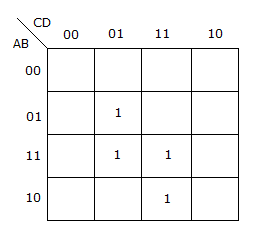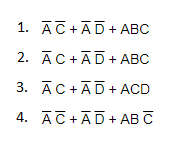Explanation: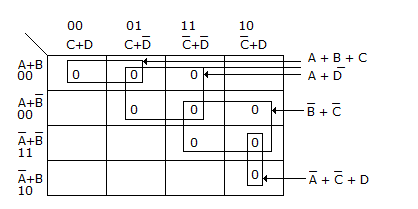Q53. A memory system of size 16 k bytes is to be designed using memory chips which have 12 address lines and 4 data lines each. The number of such chips required to design the memory system is ……….

1. 2
2. 4
3. 8
4. 18

Explanation:

(16×1024×8)/(4096×4) = 8

Q54. In a 7 segment display, LEDs b and c lit up. The decimal number displayed is ……….

1. 9
2. 7
3. 3
4. 1

Q55. In a BCD to 7 segment decoder the minimum and maximum number of outputs active at any time is ….

1. 2 and 7
2. 3 and 7
3. 1 and 6
4. 3 and 6

Explanation:

Minimum number of outputs when input is decimal 1 and maximum number of outputs when input is decimal 8.

Q56. A three state switch has three outputs. These are …….. , …….. , ……….

1. low, low and high
2. low, high, high
3. low. floating, low
4. low, high, floating

Q57. Maxterm designation for A + B + C is ……….

1. M0
2. M1
3. M3
4. M4

Explanation: A + B + C = 000 = M0

Q58. 1’s complement of 11100110 is ……………….

1. 00011001
2. 10000001
3. 00011010
4. 00000000